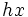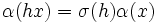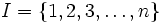# Isomorphic iff potentially conjugate in finite

## Statement

### With one isomorphism

Suppose$G$ is a finite group and$\sigma:H \to K$ is an isomorphism between two subgroups of$G$. Then, there exists a finite group$S$ containing$G$ and an element$g \in S$ such that$gxg^{-1} = \sigma(x)$ for any$x \in H$.

### With multiple isomorphisms

Suppose$G$ is a finite group and$\sigma_i:H_i \to K_i$ is a collection of isomorphisms between subgroups$H_i, K_i$ of$G$, with$i \in I$. Then, there exists a finite group$S$ containing$G$ and elements$g_i \in S$ such that$g_ixg_i^{-1} = \sigma(x)$ for any$x \in H_i$.

## Related facts

### Closely related facts

• Isomorphic iff potentially conjugate: The general version for infinite groups. Note that the proof outlined in the finite case does not work directly for infinite groups, because the finite groups version uses the fact that isomorphic subgroups have the same index.
• Equivalence of definitions of order statistics-equivalent finite groups: Two finite groups that have the same order statistics can be embedded in a symmetric group in such a way that for every conjugacy class of the symmetric group, the intersection of both groups with the conjugacy class has the same size. Conversely, if two finite groups can be embedded in any big group with this property, they have the same order statistics.

## Examples

In our examples, the finite group$L$ that we construct is much smaller than the$L$ needed for the general construction.$G$$H$ and$K$ Isomorphism class of$H,K$$L$ and embedding of$G$ in$L$ Embeddings of$H,K$ in$L$
Klein four-group any two subgroups of order two (Z2 in V4) cyclic group:Z2 dihedral group:D8, V4 in D8 non-normal subgroups of dihedral group:D8
Dihedral group:D8 center of dihedral group:D8, one of the non-normal subgroups of dihedral group:D8 cyclic group:Z2 symmetric group:S4, D8 in S4
Dihedral group:D8 the two Klein four-subgroups of dihedral group:D8 Klein four-group dihedral group:D16, D8 in D16 V4 in D16

## Proof

### With one isomorphism

Let$S$ be the symmetric group on the set$G$ with$G$ viewed as a subgroup of$S$ via the left multiplication action (this is the embedding given by Cayley's theorem). Under this embedding, the isomorphism$\sigma:H \to K$ lifts to an inner automorphism given by conjugation by a permutation$\alpha$ constructed as follows.

The restriction of$\alpha$ to$H$ is$\sigma$. Further, pick right coset representatives for$H,K$ in$G$ such that the identity element is the coset representative of the subgroups themselves. Now, define$\alpha$ as a bijection between these sets of coset representatives such that$\alpha$ sends the identity element to the identity element. Finally, for any element of the form$hx$, with$x$ a coset representative of$H$ and$h \in H$, define$\alpha(hx) = \sigma(h)\alpha(x)$.

We verify that this works. Pick$h \in H$ and an element$g \in G$. Write$g = kx$, with$x$ a coset representative of$K$ and$k \in K$. Let$l_a$ denote the left multiplication by$a$. We want to show that$l_{\sigma(h)}(kx) = \alpha(l_h(\alpha^{-1}(kx)))$.

The left side is$\sigma(h)kx$. For the right side, note that$\alpha(\sigma^{-1}(k)\alpha^{-1}(x)) = kx$, so$\alpha^{-1}(kx) = \sigma^{-1}(k)\alpha^{-1}(x)$, with$\sigma^{-1}(k) \in H$ and$\alpha^{-1}(x)$ a right coset representative of$H$. Thus, the right side is$\alpha(h\sigma^{-1}(k)\alpha^{-1}(x))$. Since$h \sigma^{-1}(k) \in H$ and$\alpha^{-1}(x)$ is a right coset representative, we get that$\alpha(h\sigma^{-1}(k)\alpha^{-1}(x)) = \sigma(h)kx$. This equals the left side, so the proof is completed.

### With multiple isomorphisms

Since$G$ is finite, so is the set$I$ of isomorphisms. Without loss of generality,$I = \{ 1,2,3,\dots,n\}$. The rough idea is that each time we take the symmetric group on the set, we can convert one isomorphism to an inner automorphism. Thus, repeating the process$n$ times yields a finite group in which all the isomorphisms are realized as inner automorphisms.

The new finite group has order$f^n(|G|)$, where$f$ is the factorial function. This grows very rapidly with$n$ for$|G| > 2$. For instance,$f^2(6)$ is$720!$ which is a number with several thousand digits.